Open Access Publications from the University of California

## Working Papers

For information about the Group in Logic and the Methodology of Science at UC Berkeley, please visit http://logic.berkeley.edu.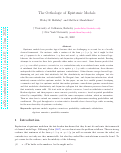## The Orthologic of Epistemic Modals

(2022)

Epistemic modals have peculiar logical features that are challenging to account for in a broadly classical framework. For instance, while a sentence of the form $p\wedge\Diamond\neg p$ ('$p$, but it might be that not $p$') appears to be a contradiction, $\Diamond \neg p$ does not entail $\neg p$, which would follow in classical logic. Likewise, the classical laws of distributivity and disjunctive syllogism fail for  epistemic modals. Existing attempts to account for these facts generally either under- or over-correct. Some theories predict that $p\wedge\Diamond\neg p$, a so-called  epistemic contradiction, is a contradiction only in an etiolated sense, under a notion of entailment that does not always allow us to replace $p\wedge\Diamond\neg p$ with a contradiction; these theories underpredict the infelicity of embedded epistemic contradictions. Other theories savage classical logic, eliminating not just rules that intuitively fail, like distributivity and disjunctive syllogism,  but also rules like non-contradiction, excluded middle, De Morgan's laws, and disjunction introduction, which intuitively remain valid for epistemic modals. In this paper, we aim for a middle ground, developing a semantics and logic for epistemic modals that makes epistemic contradictions genuine contradictions and that invalidates distributivity and disjunctive syllogism but that otherwise preserves classical laws that intuitively remain valid. We start with an algebraic semantics, based on ortholattices instead of Boolean algebras, and then propose a more concrete possibility semantics, based on partial possibilities related by compatibility. Both semantics yield the same consequence relation, which we axiomatize. Then we show how to extend our semantics to explain parallel phenomena involving probabilities and conditionals. The goal throughout is to retain what is desirable about classical logic while  accounting for the non-classicality of epistemic vocabulary.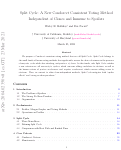## Split Cycle: A New Condorcet Consistent Voting Method Independent of Clones and Immune to Spoilers

(2021)

We introduce a new Condorcet consistent voting method, called Split Cycle. Split Cycle belongs to the small family of known voting methods satisfying independence of clones and the Pareto principle. Unlike other methods in this family, Split Cycle satisfies a new criterion we call immunity to spoilers, which concerns adding candidates to elections, as well as the known criteria of positive involvement and negative involvement, which concern adding voters to elections. Thus, relative to other clone-independent Paretian methods, Split Cycle mitigates “spoiler effects” and “strong no show paradoxes.”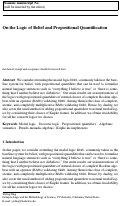## On the Logic of Belief and Propositional Quantification

(2020)

We consider extending the modal logic KD45, commonly taken as the baseline system for belief, with propositional quantifiers that can be used to formalize natural language sentences such as “everything I believe is true” or “there is some-thing that I neither believe nor disbelieve.” Our main results are axiomatizations of the logics with propositional quantifiers of natural classes of complete Boolean algebras with an operator (BAOs) validating KD45. Among them is the class of complete, atomic, and completely multiplicative BAOs validating KD45. Hence, by duality, we also cover the usual method of adding propositional quantifiers to normal modal logics by considering their classes of Kripke frames. In addition, we obtain decidability for all the concrete logics we discuss.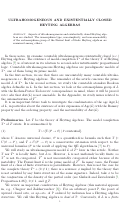## ULTRAHOMOGENEOUS AND EXISTENTIALLY CLOSED HEYTING ALGEBRAS

(2020)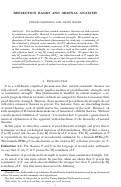## Reflection ranks and ordinal analysis

(2020)

It is well-known that natural axiomatic theories are well-ordered by consistency strength. However, it is possible to construct descending chains of artificial theories with respect to consistency strength. We provide an explanation of this well-orderness phenomenon by studying a coarsening of the consistency strength order, namely, the $\Pi^1_1$ reflection strength order. We prove that there are no descending sequences of $\Pi^1_1$ sound extensions of $\mathsf{ACA}_0$ in this order. Accordingly, we can attach a rank in this order, which we call reflection rank, to any $\Pi^1_1$ sound extension of $\mathsf{ACA}_0$. We prove that for any $\Pi^1_1$ sound theory $T$ extending $\mathsf{ACA}_0^+$, the reflection rank of $T$ equals the proof-theoretic ordinal of $T$. We also prove that the proof-theoretic ordinal of $\alpha$ iterated $\Pi^1_1$ reflection is $\varepsilon_\alpha$. Finally, we use our results to provide straightforward well-foundedness proofs of ordinal notation systems based on reflection principles.